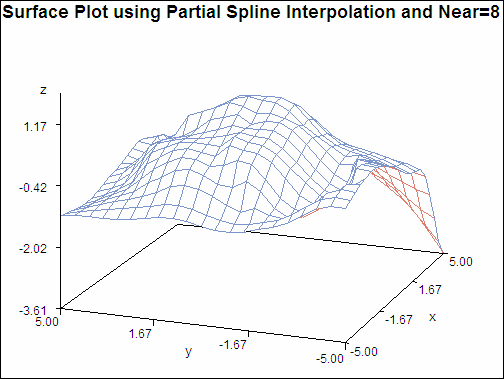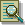The G3GRID Procedure

# Example 3: Partial Spline Interpolation

Procedure features:

GRID statement options:

 NEAR PARTIAL
Data set: NUMS (see Using the Default Interpolation Method)
Sample library member: GTGPART

This example specifies a partial spline interpolation on the GRID statement, using the eight nearest neighbors for computing the estimates of the first, and second derivatives. The output data set, when used in PROC G3D:

Surface Plot using Partial Spline Interpolation (gtgpart)`goptions reset=all border;````proc g3grid data=nums out=partial; grid y*x=z / partial near=8 axis1=-5 to 5 by .5 axis2=-5 to 5 by .5; run;````title "Surface Plot using Partial Spline Interpolation";````proc g3d data=partial; plot y*x=z; run; quit;```Previous Page | Next Page | Top of Page